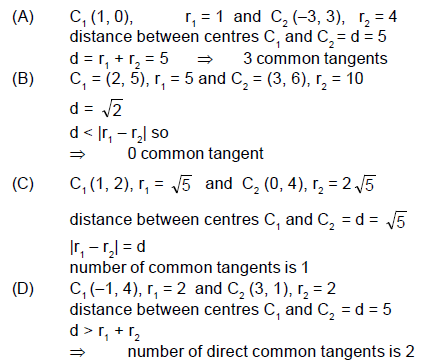Courses

# Part Test - 11 (JEE Advanced 2021)

## 72 Questions MCQ Test Mock Test Series for JEE Main & Advanced 2022 | Part Test - 11 (JEE Advanced 2021)

Description
This mock test of Part Test - 11 (JEE Advanced 2021) for JEE helps you for every JEE entrance exam. This contains 72 Multiple Choice Questions for JEE Part Test - 11 (JEE Advanced 2021) (mcq) to study with solutions a complete question bank. The solved questions answers in this Part Test - 11 (JEE Advanced 2021) quiz give you a good mix of easy questions and tough questions. JEE students definitely take this Part Test - 11 (JEE Advanced 2021) exercise for a better result in the exam. You can find other Part Test - 11 (JEE Advanced 2021) extra questions, long questions & short questions for JEE on EduRev as well by searching above.
QUESTION: 1

### A piston can freely move inside a horizontal cylinder closed from both ends. Initially, the piston separates the inside space of the cylinder into two equal parts each of  volume V0 , in which an ideal gas is contained under the same pressure p0 and at the same temperature. What work has to be performed  in order to increase isothermally the volume of one part of gas 2 times compared to that of the other by slowly moving the piston ?

Solution: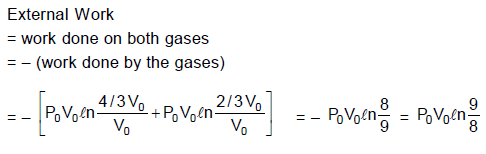QUESTION: 2

### A uniform pressure P is exerted by an external agent on all sides of a solid cube at temperature t ºC. By what amount should the temperature of the cube be raised in order to bring its volume back to its original volume before the pressure was applied if the bulk modulus is B and co-efficient of volumetric expansion is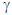?

Solution: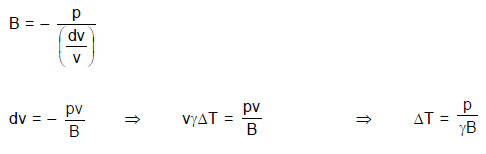QUESTION: 3

### Two rods of same dimensions, but made of different materials are joined end to end with their free ends being maintained at 100ºC and 0ºC respectively. The temperature of the junction is 70ºC. Then the temperature of the junction if the rods are interchanged will be equal to :

Solution: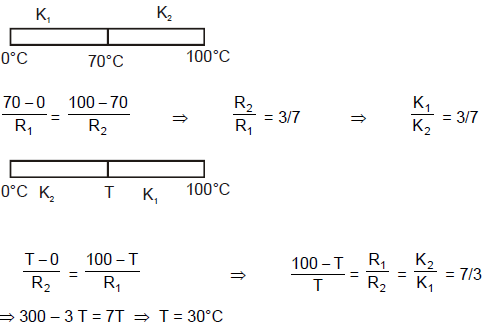QUESTION: 4

Assume a sample of an ideal gas in a vessel. Where velocity of molecules are between 2 m/sec to 5 m/sec and velocity of molecules (v) and number of molecules (n) are related as n = 7v – v2 – 10. The most probable velocity in sample is. Where v is measured in m/sec.

Solution: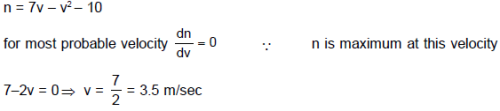QUESTION: 5

If specific heat capacity of a substance in solid and liquid state is proportional to temperature of the substance, then if heat is supplied to the solid initially at – 20°C (having melting point 0°C) at constant rate. Then the temperature dependence of substance with time will be best represented by :

Solution: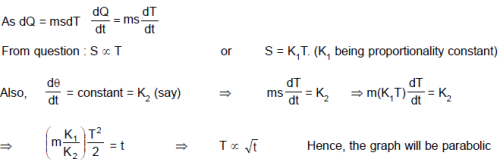QUESTION: 6

In the figure shown the pressure of the gas in state B is: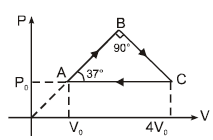Solution: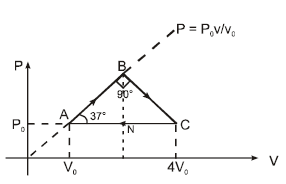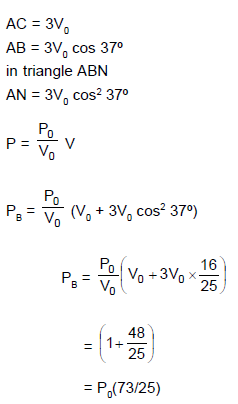QUESTION: 7

A hot black body emits the energy at the rate of 16 J m–2 s–1 and its most intense radiation corresponds to 20,000 Å. When the temperature of this body is further increased and its most intense radiation corresponds to 10,000 Å, then the energy radiated in Jm–2 s–1 will be :

Solution: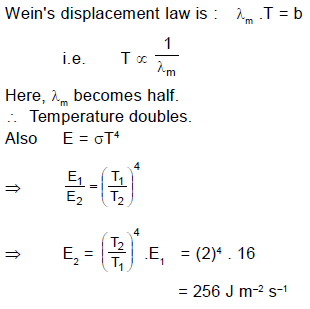QUESTION: 8

Two identical rectangular rods of metal are welded end to end in series between temperature 0°C and 100°C and 10 J of heat is conducted (in steady state process) through the rod in 2.00 min. If 5 such rods are taken and joined as shown in figure maintaining the same temperature difference between A and B,  then the time in which 20 J heat will flow through the rods  is :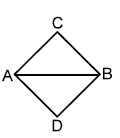Solution: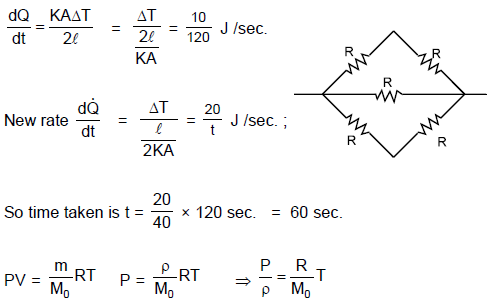*Multiple options can be correct
QUESTION: 9

An ideal gas undergoes a cyclic process abcda which is shown by pressure- density curve.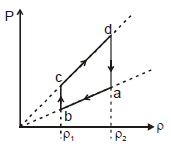Solution: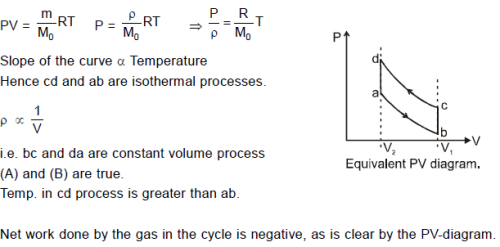*Multiple options can be correct
QUESTION: 10

The emissive power of a black body at T = 300 K is 100 Watt/m2. Consider a body B of area A = 10 m2 coefficient of reflectivity r = 0.3 and coefficient of transmission t = 0.5 and at temperature 300 K. Then which of the following is correct :

Solution: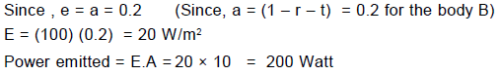*Multiple options can be correct
QUESTION: 11

The ends of a rod of uniform thermal conductivity are maintained at different (constant) temperatures. After the steady state is achieved :

Solution:

Heat obviously flows from higher temperature to lower temperature in steady state. ⇒ A is true.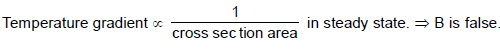Thermal current through each cross section area is same. ⇒ C is true.
Temperature decreases along the length of the rod from higher temperature end to lower temperature end. ⇒ D
is false.

*Multiple options can be correct
QUESTION: 12

Number of collisions of molecules of a gas on the wall of a container per m2 will :

Solution: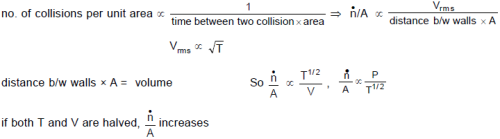QUESTION: 13

Statement -1 : It is possible for both the pressure and volume of a monoatomic ideal gas of a given amount to change simultaneously without causing the internal energy of the gas to change.

Statement-2: The internal energy of an ideal gas of a given amount remains constant if temperature does not change. It is possible to have a process in which pressure and volume are changed such that temperature remains constant.

Solution:

An isothermal process is an example to show the possibility "for both the pressure and volume of a monoatomic ideal gas to change simultaneously without causing the internal energy of the gas to change".
Hence Statement-1 is True, Statement-2 is True; Statement-2 is a correct explanation for Statement-1.

QUESTION: 14

Statement-1 : Molar heat capacity of an ideal monoatomic gas at constant volume is a constant at all temperatures.

Statement-2 : As the temperature of an monoatomic ideal gas is increased, number of degrees of freedom of gas molecules remains constant.

Solution:

For monoatomic gas the degrees of freedom are only translational and hence do not depend on temperature. Therefore molar heat capacity of an ideal monoatomic gas at constant volume is a constant at all temperatures.

QUESTION: 15

Statement-1 : Burns sustained from steam at 100ºC are usually more serious than those sustained by boiling water (at 100ºC)

Statement-2 : To convert 1gm of water at 100ºC to 1 gm of steam at 100ºC, 540 calories of heat is to be supplied. Hence 1gm of steam at 100ºC has more heat content than 1 gm of water at 100ºC

Solution:

Heat energy stored in 1 g of steam at 100°C is more in comparison to 1 g of water at 100°C. Hence burns caused by steam are more severe.

QUESTION: 16

Statement-1 : If the absolute temperature of a gas is doubled, the final rms-velocity of the gas particles becomes √2 times the initial value.
Statement-2: The average translational kinetic energy of molecules in a gas is equal to both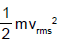and 3/2KT.( where vrms, k and T have usual meanings)

Solution: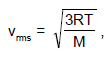if the absolute temperature T doubles, vrms must increase by √2 (m and k are constants).

QUESTION: 17

A 0.60 kg sample of water and a sample of ice are placed in two compartments A and B that are separated by a conducting wall, in a thermally insulated container. The rate of heat transfer from the water to the ice through the conducting wall is constant P, until thermal equilibrium is reached. The temperature T of the liquid water and the ice are given in graph as functions of time t. Temperature of the each compartment remain homogeneous during whole heat transfer process.
Given specific heat of ice = 2100 J/kg-K
Given specific heat of water = 4200 J/kg-K
Latent heat of fusion of ice = 3.3 × 105 J/kg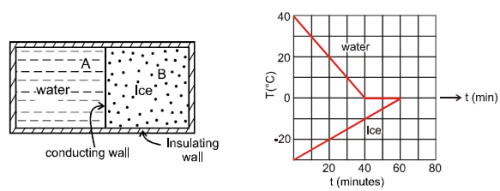Q. The value of rate P is

Solution: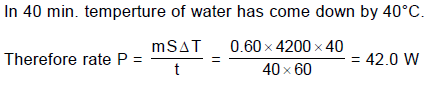QUESTION: 18

A 0.60 kg sample of water and a sample of ice are placed in two compartments A and B that are separated by a conducting wall, in a thermally insulated container. The rate of heat transfer from the water to the ice through the conducting wall is constant P, until thermal equilibrium is reached. The temperature T of the liquid water and the ice are given in graph as functions of time t. Temperature of the each compartment remain homogeneous during whole heat transfer process.
Given specific heat of ice = 2100 J/kg-K
Given specific heat of water = 4200 J/kg-K
Latent heat of fusion of ice = 3.3 × 105 J/kgQ. The initial mass of the ice in the container is equal to

Solution:

Sample of ice has been receiving heat at constant rate P from water. Its temperature has increased by 30°C in time 60 min.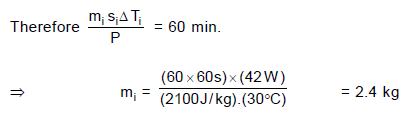QUESTION: 19

A 0.60 kg sample of water and a sample of ice are placed in two compartments A and B that are separated by a conducting wall, in a thermally insulated container. The rate of heat transfer from the water to the ice through the conducting wall is constant P, until thermal equilibrium is reached. The temperature T of the liquid water and the ice are given in graph as functions of time t. Temperature of the each compartment remain homogeneous during whole heat transfer process.
Given specific heat of ice = 2100 J/kg-K
Given specific heat of water = 4200 J/kg-K
Latent heat of fusion of ice = 3.3 × 105 J/kgQ. The mass of the ice formed due to conversion from the water till thermal equilibrium is reached, is equal to

Solution:

Thermal equilibrium reaches after 60 min. Ice conversion takes place for 20 min. During this time water at 0°C continues to give heat at rate P.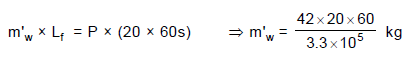= 0.15 kg

QUESTION: 20

A quantity of an ideal monoatomic gas consists of n moles initially at temperature T1. The pressure and volume both are then slowly doubled in such a manner so as to trace out a straight line on a P-V diagram.

Q. For this process, the ratio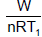is equal to (where W is work done by the gas) :

Solution: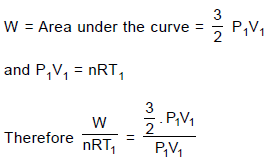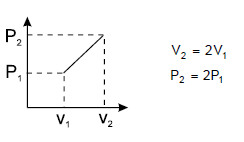QUESTION: 21

A quantity of an ideal monoatomic gas consists of n moles initially at temperature T1. The pressure and volume both are then slowly doubled in such a manner so as to trace out a straight line on a P-V diagram.

Q. For the same process, the ratio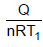is equal to (where Q is heat supplied to the gas) :

Solution: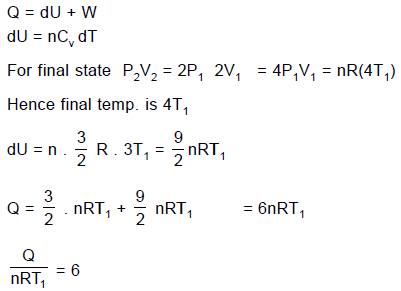QUESTION: 22

A quantity of an ideal monoatomic gas consists of n moles initially at temperature T1. The pressure and volume both are then slowly doubled in such a manner so as to trace out a straight line on a P-V diagram.

Q. If C is defined as the average molar specific heat for the process then C/R has value

Solution: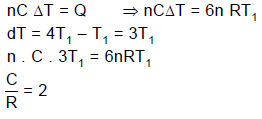QUESTION: 23

A sample of gas goes from state A to state B in four different manners, as shown by the graphs. Let W be the work done by the gas and DU be change in internal energy along the path AB. Correctly match the graphs with the statements provided.
Column I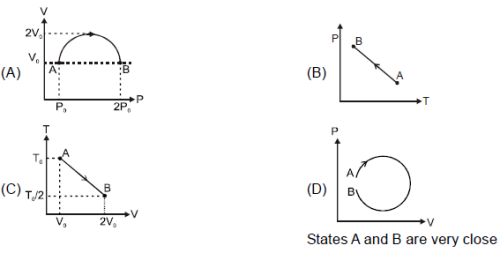Column II

(p) W is positive    (q) ΔU is negative    (r) W is negative    (s) ΔU is positive    (t) heat rejected ΔQ < 0

Solution:

In (A), V is on vertical axis.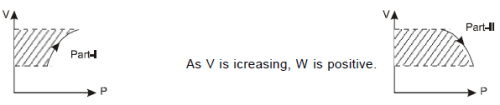As negative work in part-II is greater than positive work in part-I, net work during the process is negative.
Using PV = nRT and as Vremains same for initial and final points of the process, it is obvious that final temp.is greater than initial temperature as pressure has increased. Therefore dU is positive. Hence option (S) is
connected with (A).
Similar arguments can be applied to other graphs.

QUESTION: 24

A copper rod (initially at room temperature 20°C) of non-uniform cross section is placed between a steam chamber at 100°C and ice-water chamber at 0°C.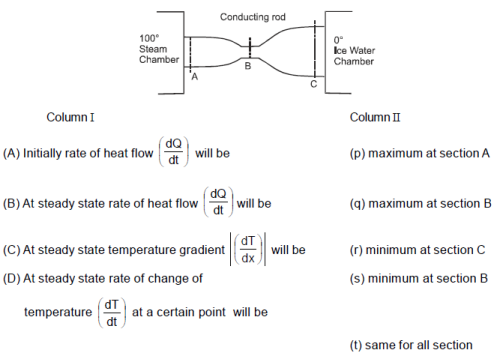Solution:

(A) Initially more heat will enter through section A but the metal will absorb some heat and less heat will
leave from C.
(B) At steady state heat accumulatron = )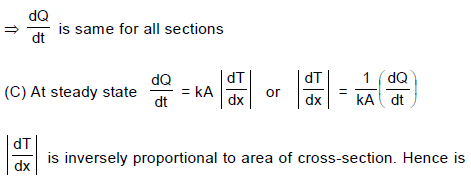maximum at B and minimum at C
(D) At steady state heat accumulation = 0
So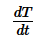=0 for any section.

QUESTION: 25

Which of the following orders is correct for the size ?

(1) Mg2+ < Na+ < F < Al     (2) Al3+ < Mg2+ < Li+ < K+    (3) Fe4+ < Fe3+ < Fe2+ < Fe    (4) Mg > Al > Si > P

Solution:

(1) Mg2+, Na+ and Fare isoelectronic and thus follows the order 12Mg2+ < 11Na+ < 9F .
Al belongs to third period and has no charge so it is largest.
Na+ = 102 pm; Mg2+ = 72 pm ; Al = 143 pm, F= 133 pm.
(2) K+ has more number of shells than Mg2+ and AI3+ . AI3+ and Mg2+ are isoelectronic but AI3+ has higher nuclear
charge so AI3+ < Mg2+. Mg2+ and Li+ has diagonal relationship. But due to +2 charge in Mg2+, the Mg2+ is smaller
than Li+. Hence Al3+ is the smallest one. K+ = 1.38 Å, Li2+ = 0.76 Å, Mg2+ = 0.72 Å and Al3+ = 0.535 Å.
(3) As the number of electrons are lost, the attraction between valence shell electrons and nucleus increases. As a
consequence of this the electrons are pulled closer to the nucleus leading to the contraction in size of ions.
(4) Across the period the nuclear charge increases and thus the size of atoms decreases. Mg = 160 pm;
Al = 143 pm; Si = 118 ; P = 110 pm.

QUESTION: 26

Which reactions involves a change in the electron–pair geometry for the under lined atoms ?

Solution: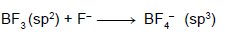QUESTION: 27

Anhydrous AlCl3 is covalent. From the data given below
Lattice Energy = 5137 KJ/mol.
ΔH hydration for Al3+ = – 4665 KJ/mol
ΔH hydration for Cl = – 381 KJ/mol
Identify the correct statement.

Solution: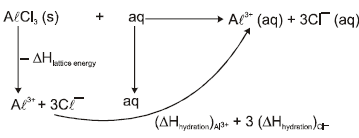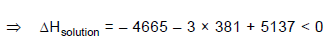Hence AlCl3 will dissolve and solution consists of hydrated Al3+ and Cl ions. In other words higher hydration
energy compensates the higher ionisation energy. As a result, it will exist as hydrated Al3+ and hydrated Cl in aqueous solution.

QUESTION: 28

In which of the following molecules / ions are all the bond angles not equal ?

Solution: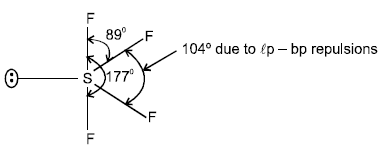Remaining molecules / ions have symmetrical structures ; so have all bonds equal.

QUESTION: 29

When sodium nitrate is heated at 800ºC :

Solution: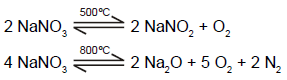QUESTION: 30

In which of the following  reaction carbon monoxide is obtained as one of the products ?

Solution: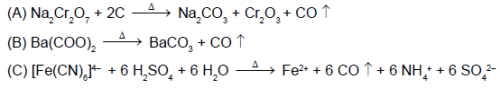QUESTION: 31

The repeating structural units in silicone is :

Solution: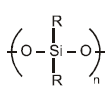QUESTION: 32

Which of the following product is obtained when boric oxide reacts with hydrogen in presence of Al or Al2Cl6?

Solution: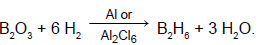*Multiple options can be correct
QUESTION: 33

Select the correct statement(s).

Solution: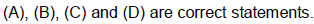*Multiple options can be correct
QUESTION: 34

Choose the correct options.

Solution:

(A) Structure is similar to that of ethane. Each N atom is tetrahedrally surrounded by
one N, two H and a lone pair. The two halves of the molecules are rotated 95º about N–N bond and occupy a gauche (non-eclipsed) conformation. The bond length is 1.45 Å.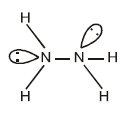(B) Has partial double bond character due to pπ-dπ delocalisation.
(C) OF2=103º (approximate) and OCl2 = 112º (approximate).

(D) Exist in polymeric structure as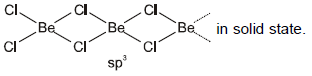*Multiple options can be correct
QUESTION: 35

In which of the following arrangements, the order(s) is/are according to the property indicated against it?

Solution:

(A) SbH3 has highest boiling point on account of highest magnitude of vander Waal's force of attraction due to highest molecular mass. Next is of NH3 due to strong H-bonding.
(B) Number of unpaired electron are 5, 4, 3 & 2 respectively.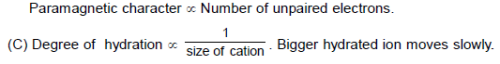D) Bond order is directly proportional to the bond energy.
Bond order of N2 = 3 , N2+ = 2.5 , N22– = 2
But N2 has more electrons in antibonding MO's and thus N2+ is more stable than N2.

*Multiple options can be correct
QUESTION: 36

Which of the following disproportionate(s) on heating with sodium hydroxide ?

Solution: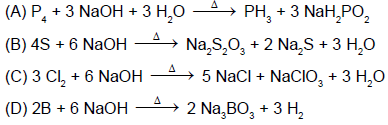QUESTION: 37

Statement-1 : In general, boiling points rise with increasing molecular weights of the hydrides of 14th group elements because

Statement-2 : The additional mass requires higher temperature for rapid movement of the molecules and the larger number of electrons in the heavier molecules provide larger London forces.

Solution:

Statement-1 and Statement-2 are True; Statement-2 is a correct explanation for Statement-1.

QUESTION: 38

Statement-1 : Bond order in a molecule can assume any value positive or negative, integral or fractional when number of electrons in bonding molecular orbitals is greater than that in anti-bonding orbitals.

Statement-2 : Bond order depends on the number of electrons in the bonding and antibonding orbitals.

Solution:

S-1 : Bond order may be a positve, whole number or fractional values not negative when NB > NA.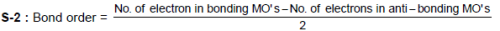QUESTION: 39

Statement - 1 : Solutions of alkali metals in liquid ammonia are good reducing agents.

Statement - 2 :  They contain free or solvated electrons.

Solution:

S-1 and S-2 are correct statements and S-2 is correct explanation of S-1.

QUESTION: 40

Statement - 1 : Grey tin becomes white on cooling to the temperature < 18ºC.

Statement - 2 :  At low temperature grey tin changes to another crystalline form, white tin.

Solution: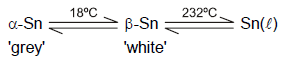QUESTION: 41

The main group elements i.e. representative elements complete their electron configuration using s and p electrons in the periodic table. These elements range from the most metallic to the most non-metallic, with intermediate properties, the semi-metals,in between. The elements which occur at the two extremes of the periodic table are highly reactive and therefore, these highly reactive elements do not occur in free state ; they usually occur in the combined forms.

Q. Tendency of I–,  Br,  CI and F to be oxidised is in order :

Solution:

Tendency to lose the electrons increases with the increase in the size of anion. (Valence shell electron(s) to be removed is farther from the nucleus in larger anion).

QUESTION: 42

The main group elements i.e. representative elements complete their electron configuration using s and p electrons in the periodic table. These elements range from the most metallic to the most non-metallic, with intermediate properties, the semi-metals,in between. The elements which occur at the two extremes of the periodic table are highly reactive and therefore, these highly reactive elements do not occur in free state ; they usually occur in the combined forms.

Q. Select the correct statement (this is with respect to the elements of main group).

Solution:

(A) Have intermediate values of ionisation energy and electron affinity.
(B) Generally, the elements found in the centre of period for main group elements are metalloids. They generally form amphoteric oxides.
(C) Down the group size of atom increases and electropositive character increases. So metallic character increases.

Across the period, size of atom decreases and electropositive character decreases. So metallic character
decreases and non-metallic character increases.

QUESTION: 43

The main group elements i.e. representative elements complete their electron configuration using s and p electrons in the periodic table. These elements range from the most metallic to the most non-metallic, with intermediate properties, the semi-metals,in between. The elements which occur at the two extremes of the periodic table are highly reactive and therefore, these highly reactive elements do not occur in free state ; they usually occur in the combined forms.

Q. Beryllium and aluminium exhibit many properties which are similar, But, the two elements differ in :

Solution:

Beryllium has the maximum vallency +2 while aluminium exhibits its valency as + 3

QUESTION: 44

Valence shell electron pair repulsion theory (VSEPR) provides a method for predicting the shape of molecules, based on the electron pair electrostatic repulsion. According to VSEPR model, molecules adopt geometries in which their valence electron pairs position themselves as far from each other as possible. The VSEPR model considers double and triple bonds to have slightly greater repulsive effects than single bonds because of the repulsive effect of π  electrons. However, the lone pair creates the maximum repulsive effect.

Q. Which species has the maximum number of lone pairs of electrons on the central atom?

Solution: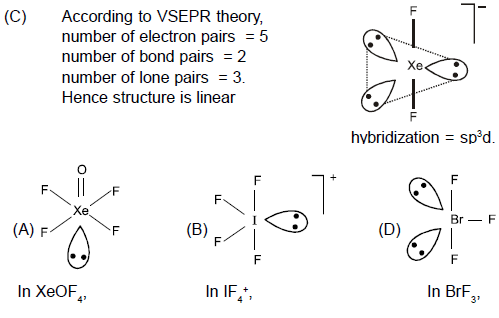QUESTION: 45

Valence shell electron pair repulsion theory (VSEPR) provides a method for predicting the shape of molecules, based on the electron pair electrostatic repulsion. According to VSEPR model, molecules adopt geometries in which their valence electron pairs position themselves as far from each other as possible. The VSEPR model considers double and triple bonds to have slightly greater repulsive effects than single bonds because of the repulsive effect of π  electrons. However, the lone pair creates the maximum repulsive effect.

Q. Incorrect order about bond angles is :

Solution: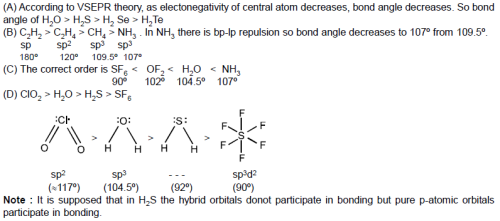QUESTION: 46

Valence shell electron pair repulsion theory (VSEPR) provides a method for predicting the shape of molecules, based on the electron pair electrostatic repulsion. According to VSEPR model, molecules adopt geometries in which their valence electron pairs position themselves as far from each other as possible. The VSEPR model considers double and triple bonds to have slightly greater repulsive effects than single bonds because of the repulsive effect of π  electrons. However, the lone pair creates the maximum repulsive effect.

Q. Which of the following does not represent the isostructural pair ?

Solution: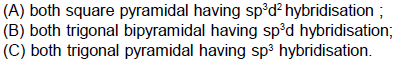QUESTION: 47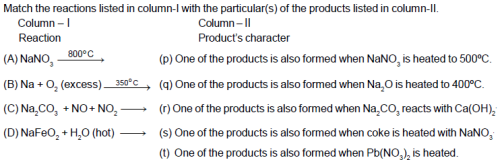Solution: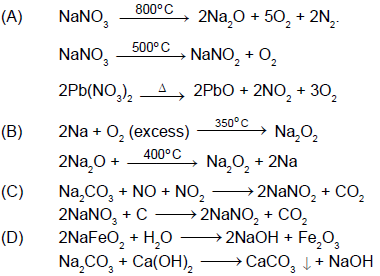QUESTION: 48

Match the group of species in Column-I with the correctly related characteristic(s) in Column-II.
Column - I                                                           Column - II

(A) CIF5 , BrF4+, IF6                           (p) All molecules/ions are polar in nature.
(B) CIF3 , BrF2+, ICI4–                                  (q) All molecules/ions have same number of lone pair(s) of electrons.
(C) XeF2 , ICI2, I3–                                     (r) All molecules/ions have same oxidation state of central atoms.
(D) CIOF3 , CIF4+, IO2F2                    (s) All molecules/ions have same hybridisation of central atoms.
(t) All molecules/ions have same shape.

Solution: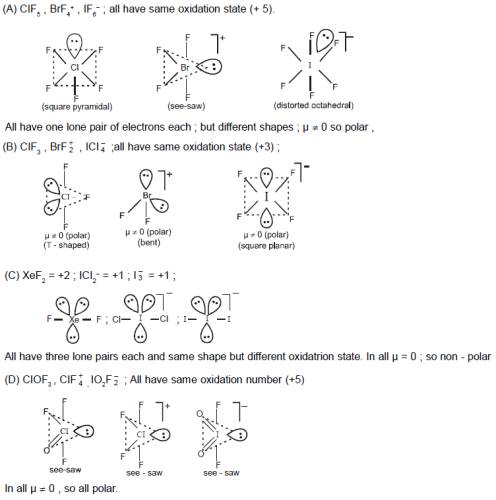QUESTION: 49

If the pairs of lines x2 + 2xy + ay2 = 0 and ax2 + 2xy + y2 = 0 have exactly one line in common then the joint equation of the other two lines is given by -

Solution: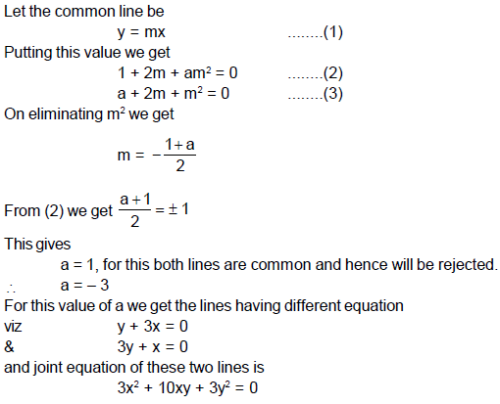QUESTION: 50

The locus of the point of intersection of the tangents to the circle x = r cos θ, y = r sinθ  at points whose parametric angles differ by π/3, is

Solution: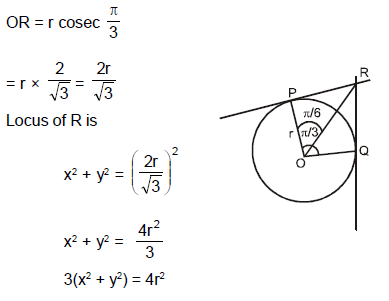QUESTION: 51

Equation of the circle for which straight lines x2 + 3y + xy + 3x = 0 are normals and size is just sufficient so that it contains the circle x2 + y2 + 2x –  6y + 9 = 0 is -

Solution: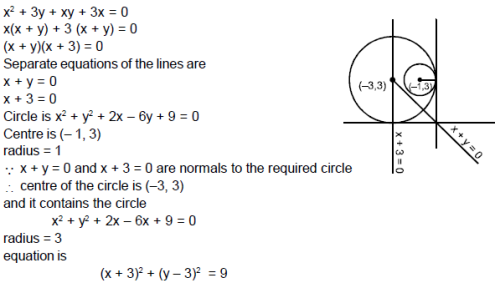QUESTION: 52

The equation of the incircle of the triangle formed by the axes and the line 4x + 3y = 6 is

Solution:
QUESTION: 53

The locus of centres of circles touching the lines x + 2y = 0 and x – 2y = 0 is

Solution:
QUESTION: 54

The equation of circumcircle of an equilateral triangle is x2 + y2 + 2gx + 2fy + c = 0 and one vertex of the triangle is (1, 1). The equation of incircle of the triangle is

Solution:
QUESTION: 55

The equation of the smallest circle passing through the points of intersection of the line x + y = 1 and the circle x2 + y2 = 9 is

Solution:
QUESTION: 56

Equation of straight line ax + by + c= 0, where 3a + 4b + c = 0, which is at maximum distance from (1, –2), is

Solution:
QUESTION: 57

If Logx (1/8) = - 3/2, then x is equal to

Solution:
QUESTION: 58

The three solutions of the equation f(x) = 0 are - 4, 8, and 11. Therefore, the three solutions of the equation f(2 x) = 0 are

Solution:
*Multiple options can be correct
QUESTION: 59

The straight lines u1 = 0, u2 = 0, u3 = 0 and u4 = 0 represent sides of a parallelogram as shown in adjacent figure, then –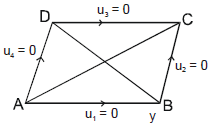Solution: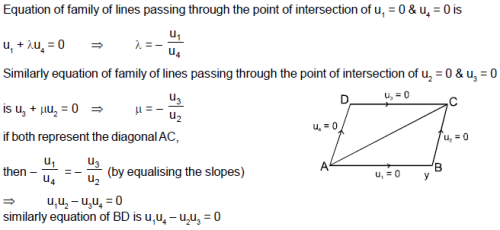QUESTION: 60

The binary operator ≠ is defined by the following truth table. Which one of the following is true about the binary operator ≠?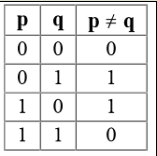Solution:
QUESTION: 61

Consider the circular region x2 + y2 = 81, What is the maximum value of the function
f(x, y) = x6 + y2(3x4 + 1) + x2.(3y4 + 1) + y6

Solution:

Rewrite the function as
f(x, y) = x2 + y2 + (x2 + y2)3
Put x2 + y2 = 81
= 81 + 813.

QUESTION: 62

Statement - 1 : The point A (3,1,6) is the mirror image of the point B(1,3,4) in the plane x–y+z=5.

Statement - 2 : The plane x – y + z = 5 bisects the line segment joining A(3, 1, 6) and B(1, 3, 4).

Solution:

Statement-1 is True, Statement-2 is True; Statement-2 is a correct explanation for Statement-1.

QUESTION: 63

Which one of the following sets of quantum numbers represents the highest energy level in an atom?

Solution:
QUESTION: 64

If f (x) = {2x - 3, x ≤ 2} {x, x < 2} then f (1) is equal to

Solution:
QUESTION: 65

In the following question, a Statement of Assertion (A) is given followed by a corresponding Reason (R) just below it. Read the Statements carefully and mark the correct answer-
Assertion(A): If M is a n x n matrix with rank n - 1, then M can be made non-singular by changing one element.
Reason(R): An n x n matrix with rank n - 1 has a non-vanishing minor of order n - 1.

Solution:
QUESTION: 66

The direction cosine of a vector a=3i+4j+5k in the direction of positive x-axis is

Solution:
QUESTION: 67

In the following question, a Statement-1 is given followed by a corresponding Statement-2 just below it. Read the statements carefully and mark the correct answer-
Consider the system of equations
ax+by=0, cx+dy=0, where a, b, c, d ∈ {0,1}
Statement-1:
The probability that the system of equations has a unique solution is 3/2 .
Statement-2:
The probability that the system of equations has a solution is 1.

Solution:
QUESTION: 68

If the polar w.r.t.y2 = 4ax touches the ellipse (x22) + (y22)=1, the locus of its pole is

Solution:
QUESTION: 69

The A.M., H.M. and G.M. between two numbers are 144/15, 15 and 12, but not necessarily in this order. Then H.M., G.M. and A.M. respectively are

Solution:
QUESTION: 70

An explosion breaks a rock into three parts in a horizontal plane. Two of them go off at right angles to each
other. The first part of mass 1 kg moves with a speed of 12 ms–1 and the second part of mass 2 kg moves with 8 ms–1 speed. If the third part files off with 4 ms–1 speed, then its mass is -

Solution:
QUESTION: 71

The eccentric angles of the extremities of the latus-rectum intersecting positive x-axis of the ellipse ((x2/a2) + (y2/b2) = 1) are given by

Solution:
QUESTION: 72Solution: